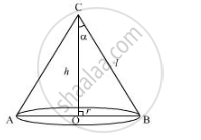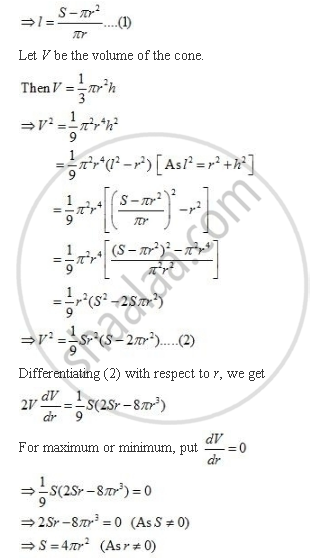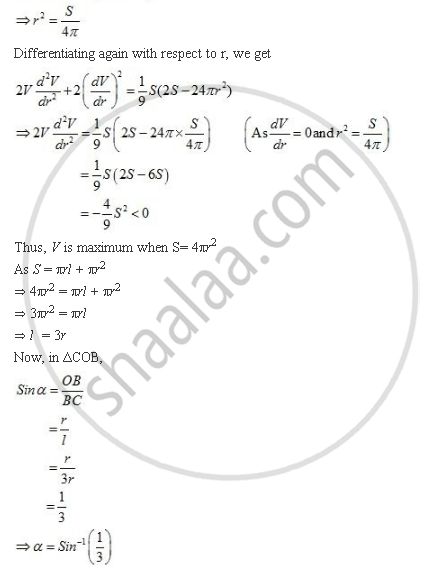Share

# Show that Semi-vertical Angle of Right Circular Cone of Given Surface Area and Maximum Volume Is Sin^(-1) (1/3) - CBSE (Commerce) Class 12 - Mathematics

#### Question

Show that semi-vertical angle of right circular cone of given surface area and maximum volume is  Sin^(-1) (1/3)

#### Solution

Let r be the radius, be the slant height and h be the height of the cone of given surface area, S.

Also, let α be the semi-vertical angle of the cone.Then πrl πr2Is there an error in this question or solution?

#### APPEARS IN

NCERT Solution for Mathematics Textbook for Class 12 (2018 to Current)
Chapter 6: Application of Derivatives
Q: 26 | Page no. 233

#### Video TutorialsVIEW ALL 

Solution Show that Semi-vertical Angle of Right Circular Cone of Given Surface Area and Maximum Volume Is Sin^(-1) (1/3) Concept: Maxima and Minima.
S